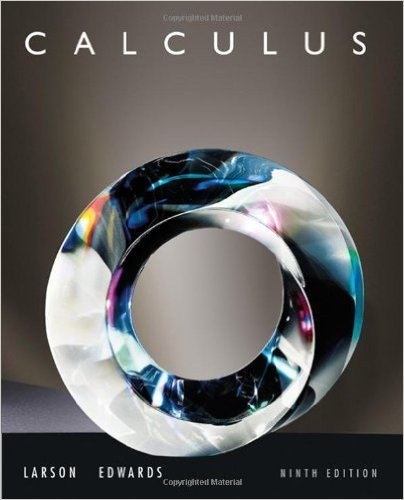×
×

# Solutions for Chapter 9.5: Alternating Series## Full solutions for Calculus | 9th Edition

ISBN: 9780547167022Solutions for Chapter 9.5: Alternating Series

Solutions for Chapter 9.5
4 5 0 335 Reviews
11
3
##### ISBN: 9780547167022

Chapter 9.5: Alternating Series includes 99 full step-by-step solutions. Calculus was written by and is associated to the ISBN: 9780547167022. Since 99 problems in chapter 9.5: Alternating Series have been answered, more than 61214 students have viewed full step-by-step solutions from this chapter. This textbook survival guide was created for the textbook: Calculus , edition: 9. This expansive textbook survival guide covers the following chapters and their solutions.

Key Calculus Terms and definitions covered in this textbook
• Central angle

An angle whose vertex is the center of a circle

• Continuous function

A function that is continuous on its entire domain

• Directed angle

See Polar coordinates.

• Divisor of a polynomial

See Division algorithm for polynomials.

• Domain of a function

The set of all input values for a function

• Double-blind experiment

A blind experiment in which the researcher gathering data from the subjects is not told which subjects have received which treatment

• Equation

A statement of equality between two expressions.

• Feasible points

Points that satisfy the constraints in a linear programming problem.

• Linear regression equation

Equation of a linear regression line

• Logarithm

An expression of the form logb x (see Logarithmic function)

• Polar distance formula

The distance between the points with polar coordinates (r1, ?1 ) and (r2, ?2 ) = 2r 12 + r 22 - 2r1r2 cos 1?1 - ?22

• Principal nth root

If bn = a, then b is an nth root of a. If bn = a and a and b have the same sign, b is the principal nth root of a (see Radical), p. 508.

• Product rule of logarithms

ogb 1RS2 = logb R + logb S, R > 0, S > 0,

• Quartic regression

A procedure for fitting a quartic function to a set of data.

• Statute mile

5280 feet.

• Stretch of factor c

A transformation of a graph obtained by multiplying all the x-coordinates (horizontal stretch) by the constant 1/c, or all of the y-coordinates (vertical stretch) of the points by a constant c, c, > 1.

• Triangular form

A special form for a system of linear equations that facilitates finding the solution.

• Triangular number

A number that is a sum of the arithmetic series 1 + 2 + 3 + ... + n for some natural number n.

• Trichotomy property

For real numbers a and b, exactly one of the following is true: a < b, a = b , or a > b.

• y-axis

Usually the vertical coordinate line in a Cartesian coordinate system with positive direction up, pp. 12, 629.

×